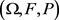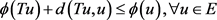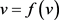﻿ 完备随机度量空间上Ekeland变分原理与Caristi不动点定理的等价性

# 完备随机度量空间上Ekeland变分原理与Caristi不动点定理的等价性Equivalence of Ekeland Variational Principle and Caristi Fixed Point Theorem in Complete Random Metric Spaces

Abstract: Firstly, it is proved that Ekeland variational principle is equivalent to Caristi fixed point theorem on complete random metric spaces under(ε,λ)-topology. Furthermore, making use of the relationship of the basic results between the two topologies, we prove that in the special random metric space—the random normed model, they are equivalent in both topologies. Finally, from Caristi fixed point theorem on complete random normed modules, the directional fixed point theorems are established on complete random normed modules under two topologies.

1. 引言

2012年，郭铁信教授与笔者建立了定义在完备随机度量空间上的Ekeland变分原理与Caristi不动点定理 。经典泛函分析中，史树中教授在文献  中研究了完备度量空间上的Ekeland变分原理与Caristi不动点定理是等价的。这启发我们在随机度量理论中来思考这个问题。虽然目前还不能确定TC-拓扑下完备随机度量空间上Ekeland变分原理与Caristi不动点定理是否等价，但本文证明了在 $\left(\epsilon ,\lambda \right)$ -拓扑下两者确实是等价的。再者，利用两种拓扑下基本结果之间的关系，本文证明了在特殊的随机度量空间——随机赋范模上，Ekeland变分原理与Caristi不动点定理在两种拓扑下都是等价的；最后由完备随机赋范模上的Caristi不动点定理，在两种拓扑下建立了完备随机赋范模上的方向压缩不动点定理。

2. 主要结果

$\left(E,d\right)$ 为以 $\left(\Omega ,F,P\right)$ 为基的 ${d}_{\epsilon ,\lambda }$ -完备RM-空间，函数 $\varphi :E\to {\stackrel{¯}{L}}^{0}\left(F\right)$ 为真的、 ${T}_{\epsilon ,\lambda }$ -下半连续的且有下界的函数。那么对于任意的 ${x}_{0}\in dom\left(\varphi \right)$，存在 $v\in dom\left(\varphi \right)$ 满足如下条件：

1) $\varphi \left(v\right)\le \varphi \left({x}_{0}\right)-d\left({x}_{0},v\right)$

2) 对于任意的 $x\in E$$x\ne v$，有 $\varphi \left(x\right)\overline{)\le }\varphi \left(v\right)-d\left(x,v\right)$ 成立，即存在 ${A}_{x}\in F$$P\left({A}_{x}\right)>0$ 使得在 ${A}_{x}$$\varphi \left(x\right)>\varphi \left(v\right)-d\left(x,v\right)$ 成立。

$\left(E,d\right)$ 为以 $\left(\Omega ,F,P\right)$ 为基的 ${d}_{\epsilon ,\lambda }$ -完备RM-空间，函数 $\varphi :E\to {\stackrel{¯}{L}}^{0}\left(F\right)$ 为真的、 ${T}_{\epsilon ,\lambda }$ -下半连续的且有下界的函数。则存在 $v\in E$ 使得 $\varphi \left(x\right)\overline{)\le }\varphi \left(v\right)-d\left(x,v\right),\forall x\ne v$

$\left(E,d\right)$ 为以为基的 ${d}_{\epsilon ,\lambda }$ -完备RM-空间，函数 $\varphi :E\to {\stackrel{¯}{L}}^{0}\left(F\right)$ 为真的、 ${T}_{\epsilon ,\lambda }$ -下半连续的且有下界的函数。映射 $T:E\to E$ 满足则T有不动点。

2) 充分性：反证法。若引理2不成立，则对于任意的 $v\in E$，存在 ${x}_{v}\in E$ 使得 ${x}_{v}\ne v$$\varphi \left({x}_{v}\right)>\varphi \left(v\right)-d\left({x}_{v},v\right)$ 成立。定义函数 $T:E\to E$$Tv={x}_{v}$。则 $Tv\ne v,\forall v\in E$$\varphi \left(Tv\right)\le \varphi \left(v\right)-d\left(Tv,v\right)$ 成立。由 $Tv\ne v,\forall v\in E$ 知，T无不动点。而由 $\varphi \left(Tv\right)\le \varphi \left(v\right)-d\left(Tv,v\right)$ 成立及引理3知T有不动点。产生矛盾，故引理2成立。

${d}_{\epsilon ,\lambda }$ -完备RM-空间上的Caristi不动点定理，可得如下 ${T}_{\epsilon ,\lambda }$ -完备RN-模上的方向压缩不动点定理。

$\left(E,‖\cdot ‖\right)$ 为数域R上以 $\left(\Omega ,F,P\right)$ 为基的 ${T}_{\epsilon ,\lambda }$ -完备RN-模。 $k\in {L}_{++}^{0}$ 且在 $\Omega$$0$T:E\to E$${T}_{\epsilon ,\lambda }$ -连续函数且具有局部性质。若对于任意的 $v\in E$，存在 ${x}_{0}\in E$ 使得 ${x}_{0}\ne v$

1) $‖v-{x}_{0}‖+‖Tv-{x}_{0}‖=‖v-Tv‖$

2) $‖Tv-{x}_{0}‖\le ‖v-{x}_{0}‖$

${\stackrel{¯}{I}}_{A}\cdot \varphi \left({\stackrel{¯}{I}}_{A}\cdot v\right)={\stackrel{¯}{I}}_{A}\cdot \frac{‖{\stackrel{¯}{I}}_{A}\cdot v-T\left({\stackrel{¯}{I}}_{A}\cdot v\right)‖}{1-k}=\frac{‖{\stackrel{¯}{I}}_{A}\cdot v-Tv‖}{1-k}={\stackrel{¯}{I}}_{A}\cdot \varphi \left(v\right),\forall A\in F$，则显然 $‖v-f\left(v\right)‖\le \varphi \left(v\right)-\varphi \left(f\left(v\right)\right)$ 成立。

$v\ne f\left(v\right)$，则 $f\left(v\right)={x}_{0}$。由(1) (2)知，$‖v-f\left(v\right)‖\le \varphi \left(v\right)-\varphi \left(f\left(v\right)\right)$ 成立。

$\left(E,‖\cdot ‖\right)$ 为数域R上以 $\left(\Omega ,F,P\right)$ 为基的TC-完备RN-模且具有可数连结性质。 $k\in {L}_{++}^{0}$ 且在 $\Omega$$0$T:E\to E$ 为TC-连续函数且具有局部性质。若对于任意的 $v\in E$，存在 ${x}_{0}\in E$ 使得 ${x}_{0}\ne v$

1) $‖v-{x}_{0}‖+‖Tv-{x}_{0}‖=‖v-Tv‖$

2) $‖Tv-{x}_{0}‖\le ‖v-{x}_{0}‖$

3. 总结

NOTES

*通讯作者。

 Guo, T.X. and Yang, Y.J. (2012) Ekeland’s Variational Principle for an -Valued Function on a Complete Random Metric Space. Journal of Mathematical Analysis and Applications, 389, 1-14.

 史树中. Ekeland 变分原理与Caristi 不动点定理的等价性[J]. 数学进展, 1987, 16(2): 203-206.

 白延琴, 熊道统. 随机度量空间上的Ekeland 变分原理、Petal定理和Drop 定理[J]. 工程数学学报, 1990, 1(7): 76-82.

 游兆永, 朱林户. E-空间上的Ekeland 变分原理[J]. 工程数学学报, 1988, 5(3): 1-7.

 Guo, T.X. (2010) Relations between Some Basic Results Derived from Two Kinds of Topologies for a Random Locally Convex Module. Journal of Functional Analysis, 258, 3024-3047.
https://doi.org/10.1016/j.jfa.2010.02.002

Top